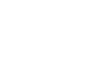What is ratio? - letsdiskussANJU M.J.

Teacher | Posted | others

# What is ratio?

0
0

Content writer | Posted

In mathematics, a ratio is a comparison of two or more numbers of the same kind indicating their sizes in relation to each other.Ratio is the number which used to express one quantity as a part of the other ones.

A ratio can be expressed as 1:2 or as a fraction ½ where the dividend that is 1 here in the example to be the antecedent and the divisor which is 2 in the example said to be the consequent.0
0

Student | Posted

Ratio is a comparison of the size of two quantities from one subject to another. It is used in mathematics, science and economics.

The most common ways we use ratio are as measurements; for example, the ratio between side lengths of a rectangle or between the age at which someone enters high school and college. Ratio can also be used to compare if two quantities are equal or unequal, to find out how much one quantity is greater than another, and to find out if something is equivalent in value to something else.0
0

translator | Posted

Ratio is how we relate two things. It compares two or more than two numbers in mathematics. It will be the size of the two numbers that are being compared. In mathematics it also indicates how many times one number is contained in the other. Ratios can be expressed in percentage or fractions.Ratios can be expressed in three ways.By using a  -colon - in between the two numbers ,by using - to- in between the numbers compared or as a fraction .0
0